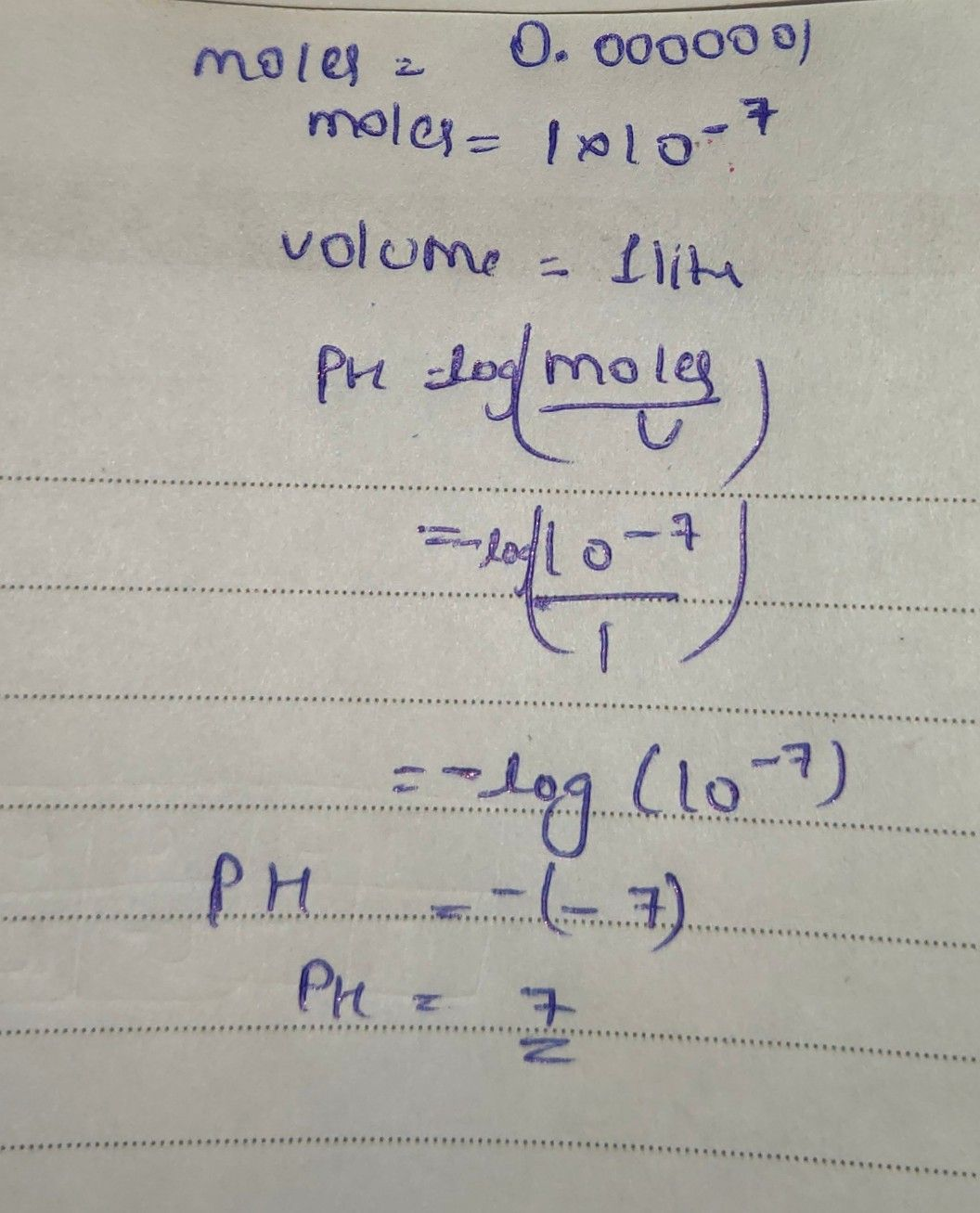Symbol
ProblemSolve the following problems. $1.A$ $1-1$ $-liter$ solution contains $.ooooo01$ moles $of$ $hy$ hydrogen ions. Find its $o1$ $leVe1$ $2.$ Suppose that an earthquake released approximately $10^{6}$ joules $ot$ eners $gy$ what is the magnitude on a Richter scale? $How$ much more energy does this earthquake release than the reference earthquake?
10th-13th grade
Trigonometry
SolutionQanda teacher - TanyaStudent
thank you